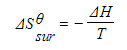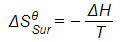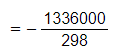# Edexcel A Level Chemistry:复习笔记5.4.2 Entropy Calculations

### Total Entropy Calculations

• If we take the reaction between sodium and chorine, we know that this is a very exothermic reaction and also involves a decrease in entropy as a solid is produced from a solid and a gas (provided a flame is used to supply the necessary activation energy)

2Na (s) + Cl2 (g) → 2NaCl (s)

• If there is a decrease in entropy, how can the reaction be spontaneous?
• We need to take into account the entropy of the surroundings as well
• The energy being released causes a substantial increase in entropy of the surroundings because there are more ways of arranging the quanta (packets of energy) in the surroundings than the system alone
• Therefore, the total entropy change for a reaction is

ΔSΘ total = ΔS Θsys + ΔSΘsurr

(sys = system and surr = surroundings)

• So, in the case of sodium and chlorine, the large amounts of energy released makes ΔSΘsurr very positive, which will outweigh the negative value of ΔS Θsys

#### Worked Example

Calculating total entropy change

Calculate the total entropy change in the formation of 1 mole of sodium chloride from its elements in their standard state

ΔSΘsys = -90.1 J K-1 mol-1

ΔSΘsurr = +1379 J K-1 mol-1

ΔS Θtotal = ΔS Θsys + ΔS Θsurr

ΔSΘ total = -90.1 + 1379 = 1289 J K-1 mol-1

### Entropy Change in the System

• Entropy changes are an order of magnitude smaller than enthalpy changes, so entropy is measured in joules rather than kilojoules. The full unit for entropy is J K-1 mol-1
• The standard entropy change (ΔSΘsystem) for a given reaction can be calculated using the standard entropies (Sꝋ ) of the reactants and products
• The equation to calculate the standard entropy change of a system is:

ΔSΘsystem = ΣΔSΘproducts - ΣΔSΘreactants

(where Σ = sum of)

• For example, the standard entropy change for the formation of ammonia (NH3) from nitrogen (N2) and hydrogen (H2) can be calculated using this equation

N2(g) + 3H2(g) ⇋ 2NH3(g)

ΔSΘsystem = (2 x ΔSΘ(NH3)) - (ΔSΘ(N2) + 3 x ΔSΘ(H2))

• Notice that, unlike enthalpy of formation for elements, entropy for elements is not zero and you can find entropy values for elements and compounds in data books

#### Worked Example

Calculating entropy changes

Calculate the entropy change of the system for the following reaction:

2Mg (s) + O2 (g) → 2MgO (s)

SΘꝋ[Mg(s)] = 32.60 J K-1 mol-1

SΘꝋ[O2(g)] = 205.0 J K-1 mol-1

SΘꝋ[MgO(s)] = 38.20 J K-1 mol-1

ΔSΘsystem = ΣΔSΘproducts - ΣΔSΘreactants

ΔSΘsystem = (2 x 38.20) - (2 x 32.60 + 205.0)

= -193.8 J K-1 mol-1

#### Worked Example

Calculating entropy changes

What is the entropy change when ammonia is formed from nitrogen and hydrogen?

N2 (g) + 3H2 (g) ⇌ 2NH3 (g)

SΘꝋ[N2 (g)] = 191.6 J K-1 mol–1

SΘꝋ[H2 (g)] = 131 J K-1 mol–1

SΘꝋ[NH3] = 192.3 J K-1 mol–1

ΔSΘsystem = ΣΔSΘproducts - ΣΔSΘreactants

ΔSΘsystem= [2 x SΘ(NH3)] - [SΘ(N2)+ (3 x SΘ(H2 ))]

ΔSΘsystem= [2 x 192.3] - [191.6 + (3 x 131)]

ΔSΘsystem = 384.6 - 584.6

ΔSΘsystem= -200 J K-1 mol–1

#### Exam Tip

Use the stoichiometry  of the equation and the correct state of the compounds when calculating the entropy change of a reaction.

### Entropy Change in the Surroundings

• To calculate the entropy change of the surroundings, ΔSΘ surr , we need to know the energy that has been transferred to them
• This is given by the enthalpy change, ΔH, and the relationship can be expressed as:• T is the absolute temperature
• The entropy change of the surroundings depends upon temperature
• The transfer of a given quantity of energy to surroundings at a low temperature will produce a greater entropy change than the transfer of the same amount of energy to the surroundings at a higher temperature

#### Worked Example

Calculating entropy of surroundings

Calculating entropy of surroundings for the reaction between aluminium oxide and carbon at 298 K

Al2O3 (s) + 3C (s) → 2Al (s) + 3CO (g)

ΔHΘ = +1336 kJ mol-1

T = 298 K

••